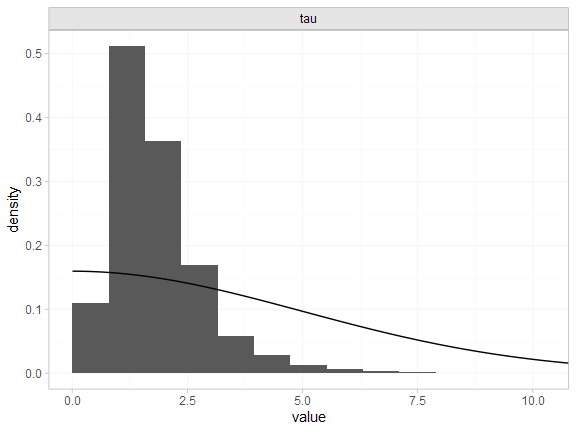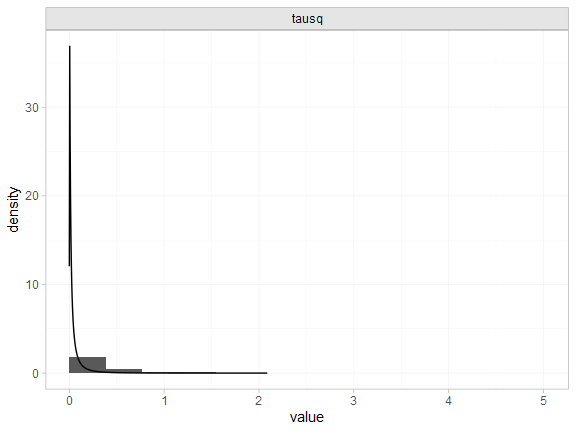# Example: White blood cell transfusion

library(multinma)
options(mc.cores = parallel::detectCores())
#> For execution on a local, multicore CPU with excess RAM we recommend calling
#> options(mc.cores = parallel::detectCores())
#>
#> Attaching package: 'multinma'
#> The following objects are masked from 'package:stats':
#>
#>     dgamma, pgamma, qgamma

This vignette describes the analysis of 6 trials comparing transfusion of granulocytes (white blood cells) to control for preventing mortality in patients with neutropenia or neutrophil dysfunction (Stanworth et al. 2005; Turner et al. 2012). The data are available in this package as transfusion:

head(transfusion)
#>        studyc        trtc  r  n
#> 1    Bow 1984 Transfusion  5 13
#> 2    Bow 1984     Control  4 11
#> 3 Herzig 1977 Transfusion  1 13
#> 4 Herzig 1977     Control  3 14
#> 5  Higby 1975 Transfusion  2 17
#> 6  Higby 1975     Control 14 19

Turner et al. (2012) previously used this dataset to demonstrate the application of informative priors for heterogeneity, an analysis which we recreate here.

### Setting up the network

We begin by setting up the network - here just a pairwise meta-analysis. We have arm-level count data giving the number of deaths (r) out of the total (n) in each arm, so we use the function set_agd_arm(). We set “Control” as the reference treatment.

tr_net <- set_agd_arm(transfusion,
study = studyc,
trt = trtc,
r = r,
n = n,
trt_ref = "Control")
tr_net
#> A network with 6 AgD studies (arm-based).
#>
#> ------------------------------------------------------- AgD studies (arm-based) ----
#>  Study         Treatments
#>  Bow 1984      2: Transfusion | Control
#>  Herzig 1977   2: Transfusion | Control
#>  Higby 1975    2: Transfusion | Control
#>  Scali 1978    2: Transfusion | Control
#>  Vogler 1977   2: Transfusion | Control
#>  Winston 1982a 2: Transfusion | Control
#>
#>  Outcome type: count
#> ------------------------------------------------------------------------------------
#> Total number of treatments: 2
#> Total number of studies: 6
#> Reference treatment is: Control
#> Network is connected

### Meta-analysis models

We fit two random effects models, first with a non-informative prior for the heterogeneity, then using the informative prior described by Turner et al. (2012).

#### Random effects meta-analysis with non-informative heterogeneity prior

We fit a random effects model using the nma() function with trt_effects = "random". We use $$\mathrm{N}(0, 100^2)$$ prior distributions for the treatment effects $$d_k$$ and study-specific intercepts $$\mu_j$$, and a non-informative $$\textrm{half-N}(5^2)$$ prior for the heterogeneity standard deviation $$\tau$$. We can examine the range of parameter values implied by these prior distributions with the summary() method:

summary(normal(scale = 100))
#> A Normal prior distribution: location = 0, scale = 100.
#> 50% of the prior density lies between -67.45 and 67.45.
#> 95% of the prior density lies between -196 and 196.
summary(half_normal(scale = 5))
#> A half-Normal prior distribution: location = 0, scale = 5.
#> 50% of the prior density lies between 0 and 3.37.
#> 95% of the prior density lies between 0 and 9.8.

Fitting the RE model

tr_fit_RE_noninf <- nma(tr_net,
trt_effects = "random",
prior_intercept = normal(scale = 100),
prior_trt = normal(scale = 100),
prior_het = half_normal(scale = 5))

Basic parameter summaries are given by the print() method:

tr_fit_RE_noninf
#> A random effects NMA with a binomial likelihood (logit link).
#> Inference for Stan model: binomial_1par.
#> 4 chains, each with iter=2000; warmup=1000; thin=1;
#> post-warmup draws per chain=1000, total post-warmup draws=4000.
#>
#>                   mean se_mean   sd    2.5%     25%     50%     75%   97.5% n_eff Rhat
#> d[Transfusion]   -1.13    0.02 0.96   -3.26   -1.64   -1.07   -0.58    0.66  1506    1
#> lp__           -134.41    0.10 3.20 -141.87 -136.22 -134.01 -132.19 -129.17  1074    1
#> tau               1.83    0.03 1.03    0.52    1.14    1.60    2.27    4.50  1383    1
#>
#> Samples were drawn using NUTS(diag_e) at Thu Nov 26 10:15:37 2020.
#> For each parameter, n_eff is a crude measure of effective sample size,
#> and Rhat is the potential scale reduction factor on split chains (at
#> convergence, Rhat=1).

By default, summaries of the study-specific intercepts $$\mu_j$$ and study-specific relative effects $$\delta_{jk}$$ are hidden, but could be examined by changing the pars argument:

# Not run
print(tr_fit_RE_noninf, pars = c("d", "mu", "delta"))

The prior and posterior distributions can be compared visually using the plot_prior_posterior() function:

plot_prior_posterior(tr_fit_RE_noninf, prior = "het")The posterior distribution for the heterogeneity variance $$\tau^2$$ is summarised by

noninf_tau <- as.array(tr_fit_RE_noninf, pars = "tau")
noninf_tausq <- noninf_tau^2
names(noninf_tausq) <- "tausq"
summary(noninf_tausq)
#>       mean   sd 2.5%  25%  50%  75% 97.5% Bulk_ESS Tail_ESS Rhat
#> tausq  4.4 6.13 0.27 1.31 2.55 5.13 20.27     1250     1539    1

#### Random effects meta-analysis with informative heterogeneity prior

Keeping the rest of the model setup the same, we now use an informative $$\textrm{log-N}(-3.93, 1.51^2)$$ prior for the heterogeneity variance $$\tau^2$$. We can examine the range of parameter values implied by this prior distribution with the summary() method:

summary(log_normal(-3.93, 1.51))
#> A log-Normal prior distribution: location = -3.93, scale = 1.51.
#> 50% of the prior density lies between 0.01 and 0.05.
#> 95% of the prior density lies between 0 and 0.38.

Fitting the RE model, we specify the log_normal prior distribution in the prior_het argument, and set prior_het_type = "var" to indicate that this prior distribution is on the variance scale (instead of the standard deviation, the default).

tr_fit_RE_inf <- nma(tr_net,
trt_effects = "random",
prior_intercept = normal(scale = 100),
prior_trt = normal(scale = 100),
prior_het = log_normal(-3.93, 1.51),
prior_het_type = "var")

Basic parameter summaries are given by the print() method:

tr_fit_RE_inf
#> A random effects NMA with a binomial likelihood (logit link).
#> Inference for Stan model: binomial_1par.
#> 4 chains, each with iter=2000; warmup=1000; thin=1;
#> post-warmup draws per chain=1000, total post-warmup draws=4000.
#>
#>                   mean se_mean   sd    2.5%     25%     50%     75%   97.5% n_eff Rhat
#> d[Transfusion]   -0.78    0.01 0.43   -1.69   -1.04   -0.75   -0.48   -0.01  2157    1
#> lp__           -140.17    0.07 2.81 -146.56 -141.90 -139.88 -138.14 -135.65  1457    1
#> tau               0.48    0.01 0.34    0.05    0.21    0.43    0.69    1.30  1469    1
#>
#> Samples were drawn using NUTS(diag_e) at Thu Nov 26 10:16:02 2020.
#> For each parameter, n_eff is a crude measure of effective sample size,
#> and Rhat is the potential scale reduction factor on split chains (at
#> convergence, Rhat=1).

By default, summaries of the study-specific intercepts $$\mu_j$$ and study-specific relative effects $$\delta_{jk}$$ are hidden, but could be examined by changing the pars argument:

# Not run
print(tr_fit_RE_inf, pars = c("d", "mu", "delta"))

The prior and posterior distributions can be compared visually using the plot_prior_posterior() function:

plot_prior_posterior(tr_fit_RE_inf, prior = "het")Note: The heterogeneity variance $$\tau^2$$ is plotted here since the prior was specified on $$\tau^2$$.

The posterior distribution for the heterogeneity variance $$\tau^2$$ is summarised by

inf_tau <- as.array(tr_fit_RE_inf, pars = "tau")
inf_tausq <- inf_tau^2
names(inf_tausq) <- "tausq"
summary(inf_tausq)
#>       mean   sd 2.5%  25%  50%  75% 97.5% Bulk_ESS Tail_ESS Rhat
#> tausq 0.35 0.48    0 0.04 0.18 0.47  1.69     1422     2566    1